Math Portal Home > Middle School Lesson Plans > Spaghetti Bridges: Teacher Notes

Spaghetti Bridges: Teacher Notes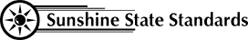MA.8.S.3.1
MA.912.A.3.11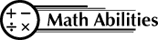Conceptual Understanding

Data Collection
Graphing
Linear Equations
Interpret Data

Procedural Knowledge

Organize Data
Graph Data
Make Predictions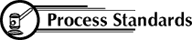Problem Solving
Reasoning
Communication
Connections
RepresentationEngineers test the materials used in construction of buildings, roads, bridges, etc., for durability, strength, and safety. Testing models gives them the information in a manageable, cost efficient manner. The following activity is similar to a procedure used in testing the strength of bridge beams.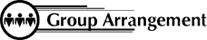Students work in pairs.Each pair needs:

• 1 paper cup with 2 holes punched on opposite sides
• 1 cupful of pennies (about 100)
• Uncooked spaghetti (about 25 pieces)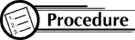1. Thread a piece of spaghetti through the holes in the cup.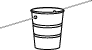2. One person will suspend the cup by placing each index finger approximately one inch in from the ends of the spaghetti.
3. Another student will carefully add pennies to the cup, one at a time, until the spaghetti breaks.
4. Record results below.
5. Repeat the above procedure with 2, 3, 4, and 5 pieces of spaghetti until one or more pieces of spaghetti breaks.

 (x) (Independent Variable) # of spaghetti (y) (Dependent Variable) # of pennies 1 2 3 4 5

6. GRAPH THE RESULTS: Plot the data from your table on a coordinate plane as ordered pairs (x, y).
7. READ THE RESULTS: Looking at your graphed points, do they seem to lie along a straight line or curve?

Use the graph to answer the following:

1. Find the number of pennies needed to break "bridges" of 6 pieces: _____ pennies, 7 pieces: ______ pennies, 8 pieces: ______ pennies.

2. About how many more pennies are required to break a "bridge" each time another piece of spaghetti was added? How can you tell this from your graph?

3. Extend the line or curve you have drawn so that it crosses the y-axis. Identify the ordered pair where this occurs: (0, ___ ). It sounds crazy, but the graph may suggest that a bridge of no spaghetti would crumple with a weight of ______ pennies.

8. Describe in words how to determine the number of pennies needed to break a bridge if you know the number of pieces of spaghetti.

9. Use your description to predict how many pennies would be needed before a bridge of 20 pieces of spaghetti would break. Show your work!

10. Translate your words above to an equation that could be used to determine the number of pennies (y) needed if you know the number of pieces of spaghetti (x).

y =

11. Use your equation to predict the number of pennies needed to break a bridge of 50 pieces of spaghetti.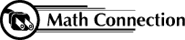As a result of this activity, students will be able to collect, graph and interpret data.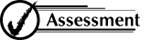Have students write answers to the following questions in their journals:

1. Explain how they knew the graph was linear.
2. Using the equation, find the number of pennies needed to break a bridge of 75 pieces of spaghetti.

 Math Portal Home > Middle School Lesson Plans > Spaghetti Bridges: Teacher Notes